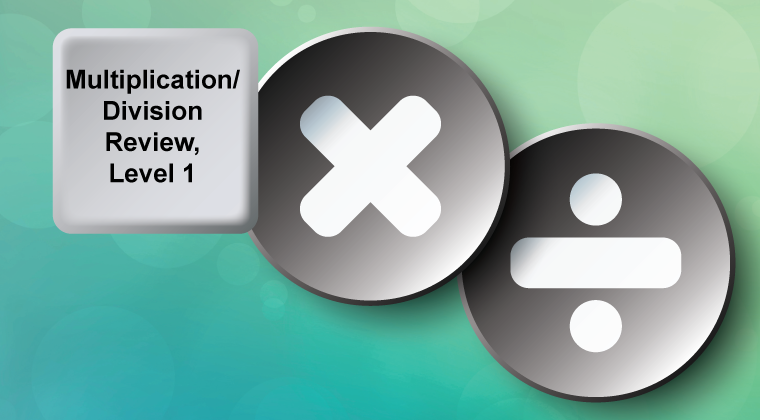## Course curriculum

1. Lesson 1 Overview

2. Multiplication and Division Terms

3. Slide Show: Math Vocabulary

4. Math Vocabulary Word Search Puzzle (Interactive)

5. Math Vocabulary Crossword Puzzle (Interactive)

6. Lesson 1 Quiz

1. Lesson 2 Overview

2. Tutorial: Fact Families, Multiplication and Division

3. Interactive Math Game: Fact Families, Multiplication and Division

4. Worksheet: Fact Families

5. Lesson 2 Quiz

1. Lesson 3 Overview

2. Tutorial: Column Multiplication

3. Video Tutorial: Numbers in Expanded Form

4. Slide Show: Math Examples: Column Multiplication

5. Worksheet: Multiplying Two-Digit Numbers by One-Digit Numbers

6. Worksheet: Multiplying Two-Digit Numbers by One-Digit Numbers

7. Lesson 3 Quiz

1. Lesson 4 Overview

2. Tutorial: Divisibility Rules

3. Interactive Math Game: Divisibility Circus (Divisibility by 2)

4. Interactive Math Game: Divisibility Circus (Divisible by 3)

5. Interactive Math Game: Divisibility Circus (Divisible by 4)

6. Interactive Math Game: Divisibility Circus (Divisible by 5)

7. Interactive Math Game: Divisibility Circus (Divisible by 8)

8. Interactive Math Game: Divisibility Circus (Divisible by 9)

9. Worksheet: Divisibility Rules

10. Lesson 4 Quiz

1. Lesson 5 Overview

2. Tutorial: Ways of Dividing

3. Tutorial: Long Division

4. Slide Show: Math Examples: Long Division

5. Worksheet: Long Division

6. Worksheet: Long Division 2

7. Lesson 5 Quiz